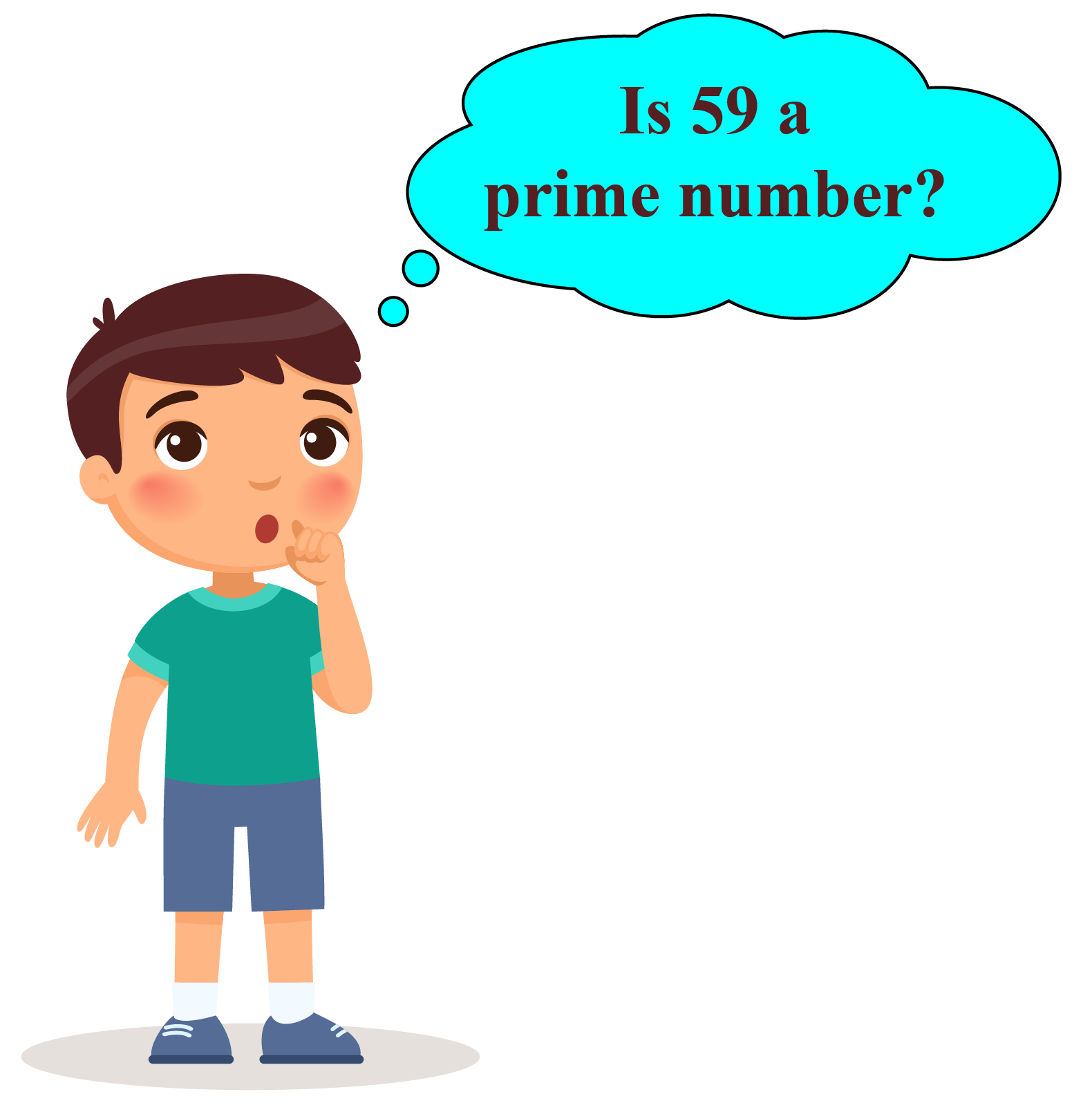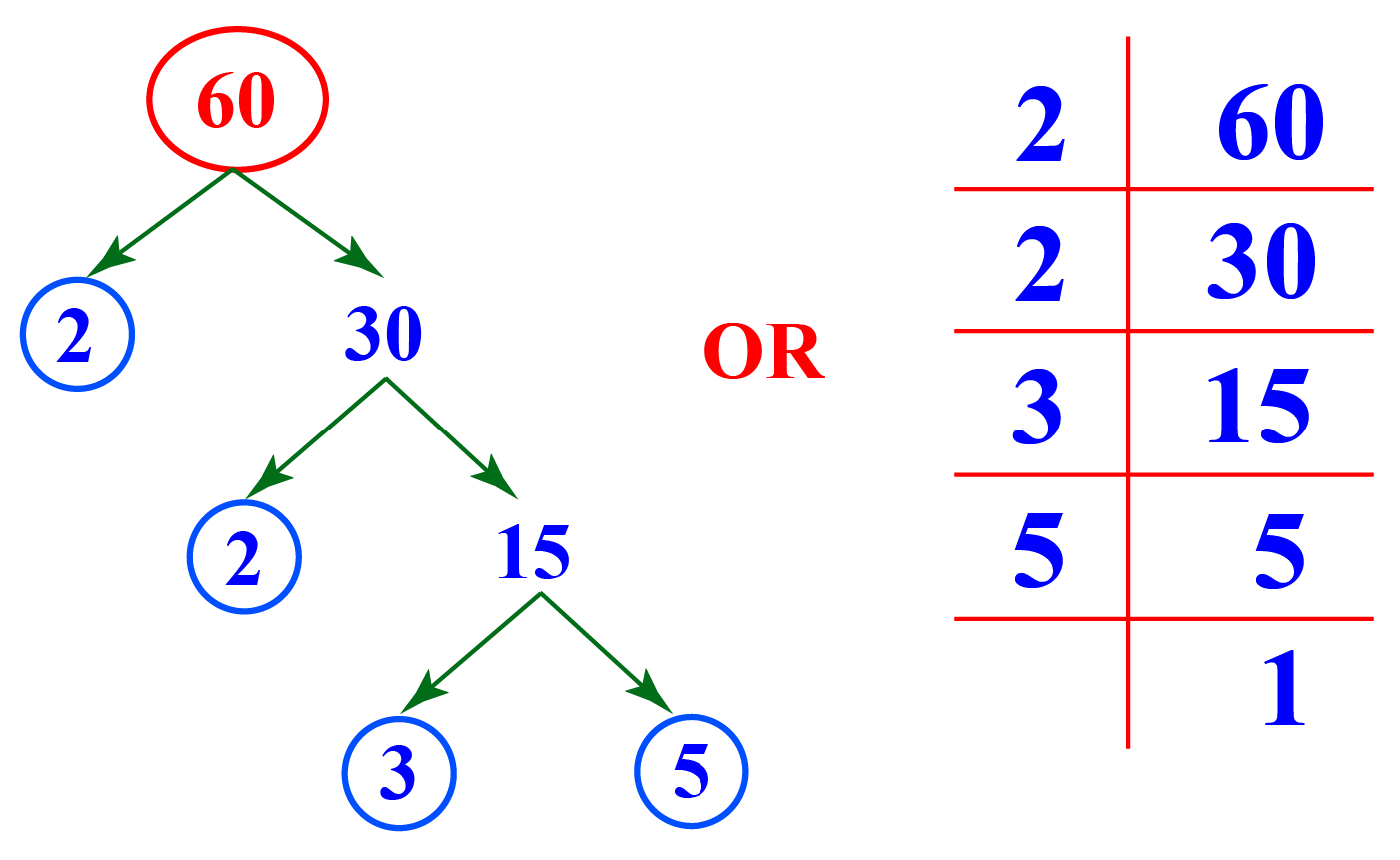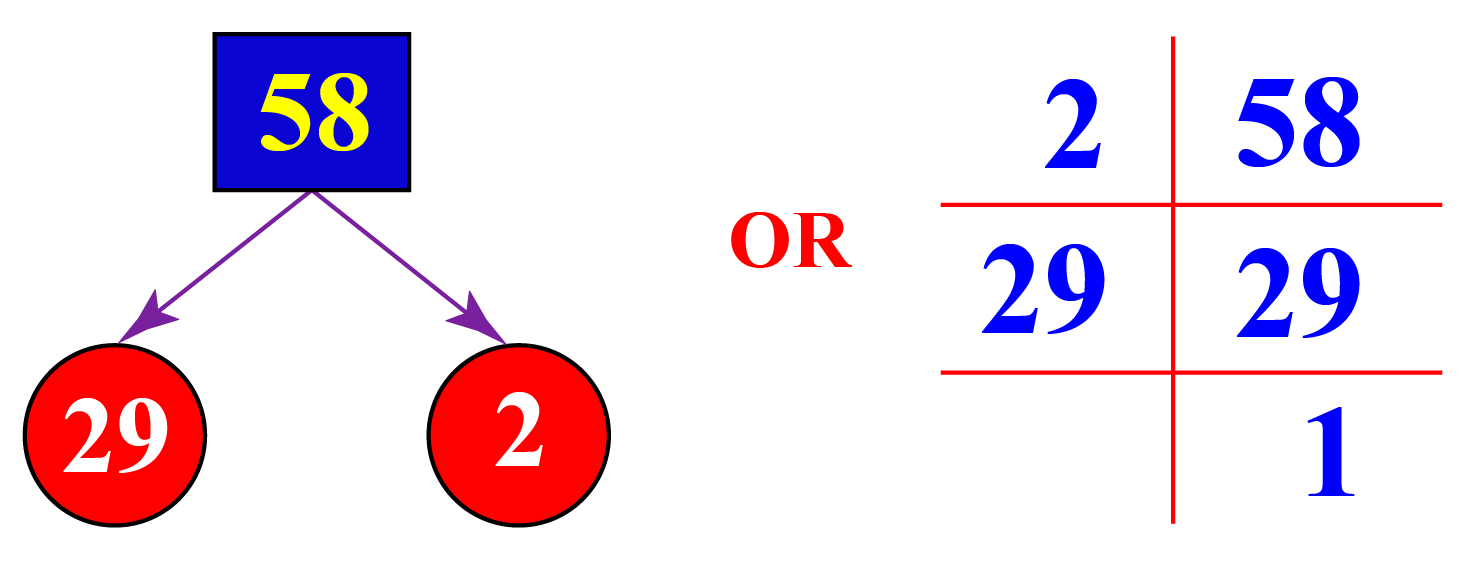# Is 59 a Prime Number

Is 59 a Prime Number
Go back to  'Prime Numbers'

Did you know that the first written evidence of prime numbers was found in 1550 BC?

If a number cannot be divided into equal groups, then it is known as a prime number.

Can you divide 59 into equal groups of 2? equal groups of 3 ? groups of 4?Let's find an answer to this question!

In this mini-lesson, we will explore the world of prime numbers by finding the answers to the questions like what is a prime number, is 59 a prime number, or if  59 is a composite number, etc., with the help of solved examples and interactive questions.

## What Is a Prime Number?

Any whole number greater than 1, having only 2 factors, 1 and the number itself is known as a prime number.

For example; 23 is a prime number because it has only 2 factors 1 and the number itself, i.e. 23

## Is 59 a Prime Number?

To determine if 59 is a prime number, let us see the factor tree of 59

59 has only two factors, that is, 1 and 59

This implies, 59 is a prime number as it is factored only as 1 and the number itself i.e. 59

The very first thing that one can take note of is, 59 is an odd number.

2 is the only even number which is prime, all other prime numbers are odd.

But what about the number 91?

Is 91 a prime number?

## Solved Examples

 Example 1

Lucy has to list prime numbers between 70 and 100. Help her list them.

Solution

Lucy listed the following prime numbers between 70 and 100. They are:

71, 73, 79, 83, 89 and 97

She found there were 6 prime numbers between 70 to 100

 $$\therefore$$ Lucy listed 6 prime numbers between 70 and 100
 Example 2

Eric has to prove that 1 added or subtracted in 59, makes it a composite number. How will he prove it?

Solution

On adding of 1 to 59, Eric will get, 60. On subtracting 1 from 59, he will get 58

He will check whether 60 is a prime number or not.

He will check the divisibility of 60 by 2, 3, 5, and 7

Or he will simply find out prime factorization of 60Factors of 60 are, 1, 2, 3, 4, 5, 6, 10, 12, 15, 20, 30, 60

It has factors other than 1 and 60, which makes it a composite number.

He will check whether 58 is a prime number.

He will check the divisibility of 58 by 2, 3, 5, and 7

Or he will simply find out prime factorization of 58Factors of 58 are 1, 29, and 58

It has factors other than 1 and 58, which makes it a composite number.

Hence 1 added or subtracted in 59, makes it a composite number.

 Hence Proved

## Interactive Questions

Here are a few activities for you to practice.Challenging Questions
• Find out prime numbers from 1 to 100 whose sum of digits is also a prime number.
• How can you arrange 53 blocks in rows such that each row has an equal number of blocks? Based on their arrangement check- is 53 a prime number?

## Let's Summarize

We hope you enjoyed learning about the number 59. Now that you know it is a prime number, you will be able to easily solve problems on prime numbers, is 91 a prime number and is 53 a prime number.

At Cuemath, our team of math experts is dedicated to making learning fun for our favorite readers, the students!

Through an interactive and engaging learning-teaching-learning approach, the teachers explore all angles of a topic.

Be it worksheets, online classes, doubt sessions, or any other form of relation, it’s the logical thinking and smart learning approach that we, at Cuemath, believe in.

## 1. What are co-prime numbers?

It is a set of any two numbers which have only 1 as their common factor. For example, 5 and 6 are co-prime numbers.

## 2. What is the prime factorization of 59?

The prime factorization of 59 is the product of prime factors of 59 which result in 59. The prime factorization of 59 is $$1 \times 59$$..

## 3. Is 59 a prime number? Yes or No?

Yes, 59 is a prime number.

More Important Topics
Numbers
Algebra
Geometry
Measurement
Money
Data
Trigonometry
Calculus